# Discrete-Time Integrator

Perform discrete-time integration or accumulation of signal

• Library:
• Simulink / Commonly Used Blocks

HDL Coder / Discrete

HDL Coder / HDL Floating Point Operations

•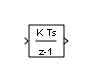## Description

Use the Discrete-Time Integrator block in place of the Integrator block to create a purely discrete model. With the Discrete-Time Integrator block, you can:

• Define initial conditions on the block dialog box or as input to the block

• Define an input gain (K) value

• Output the block state

• Define upper and lower limits on the integral

• Reset the state with an additional reset input

### Output Equations

With the first time step, block state `n = 0`, with either initial output `y(0) = IC` or initial state `x(0) = IC`, depending on the Initial condition setting parameter value.

For a given step `n > 0` with simulation time `t(n)`, Simulink® updates output `y(n)` as follows:

• Forward Euler method:

`y(n) = y(n-1) + K*[t(n) - t(n-1)]*u(n-1)`
• Backward Euler method:

`y(n) = y(n-1) + K*[t(n) - t(n-1)]*u(n)`
• Trapezoidal method:

`y(n) = y(n-1) + K*[t(n)-t(n-1)]*[u(n)+u(n-1)]/2`

Simulink automatically selects a state-space realization of these output equations depending on the block sample time, which can be explicit or triggered. When using explicit sample time, `t(n)-t(n-1)` reduces to the sample time `T` for all `n > 0`.

### Integration and Accumulation Methods

This block can integrate or accumulate a signal using a forward Euler, backward Euler, or trapezoidal method. Assume that `u` is the input, `y` is the output, and `x` is the state. For a given step `n`, Simulink updates `y(n)` and `x(n+1)`. In integration mode, `T` is the block sample time (delta `T` in the case of triggered sample time). In accumulation mode, `T = 1`. The block sample time determines when the output is computed but not the output value. `K` is the gain value. Values clip according to upper or lower limits.

Forward Euler Method

Forward Euler method (default), also known as forward rectangular, or left-hand approximation

The software approximates `1/s` as `T/(z-1)`. The expressions for the output of the block at step `n` are:

```x(n+1) = x(n) + K*T*u(n) y(n) = x(n)```

The block uses these steps to compute the output:

```Step 0: y(0) = IC (clip if necessary) x(1) = y(0) + K*T*u(0) Step 1: y(1) = x(1) x(2) = x(1) + K*T*u(1) Step n: y(n) = x(n) x(n+1) = x(n) + K*T*u(n) (clip if necessary)```

Using this method, input port 1 does not have direct feedthrough.

Backward Euler Method

Backward Euler method, also known as backward rectangular or right-hand approximation

The software approximates `1/s` as `T*z/(z-1)`. The resulting expression for the output of the block at step `n` is

`y(n) = y(n-1) + K*T*u(n).`

Let `x(n) = y((n)-1)`. The block uses these steps to compute the output.

• If the parameter Initial condition setting is set to `Output` or `Auto` for triggered and function-call subsystems:

```Step 0: y(0) = IC (clipped if necessary) x(1) = y(0)```
• If the parameter Initial condition setting is set to `Auto` for non-triggered subsystems:

```Step 0: x(0) = IC (clipped if necessary) x(1) = y(0) = x(0) + K*T*u(0) Step 1: y(1) = x(1) + K*T*u(1) x(2) = y(1) Step n: y(n) = x(n) + K*T*u(n) x(n+1) = y(n)```

Using this method, input port 1 has direct feedthrough.

Trapezoidal Method

For this method, the software approximates `1/s` as `T/2*(z+1)/(z-1)`.

When `T` is fixed (equal to the sampling period), the expressions to compute the output are:

```x(n) = y(n-1) + K*T/2*u(n-1) y(n) = x(n) + K*T/2*u(n)```
• If the parameter Initial condition setting is set to `Output` or `Auto` for triggered and function-call subsystems:

```Step 0: y(0) = IC (clipped if necessary) x(1) = y(0) + K*T/2*u(0)```
• If the parameter Initial condition setting is set to `Auto` for non-triggered subsystems:

```Step 0: x(0) = IC (clipped if necessary) y(0) = x(0) + K*T/2*u(0) x(1) = y(0) + K*T/2*u(0) Step 1: y(1) = x(1) + K*T/2*u(1) x(2) = y(1) + K*T/2*u(1) Step n: y(n) = x(n) + K*T/2*u(n) x(n+1) = y(n) + K*T/2*u(n)```

Here, `x(n+1)` is the best estimate of the next output. It is not the same as the state, in that `x(n)` is not equal to `y(n)`.

Using this method, input port 1 has direct feedthrough.

When T is a Variable

When`T` is a variable (for example, obtained from the triggering times), the block uses these steps to compute the output.

• If the parameter Initial condition setting is set to `Output` or `Auto` for triggered and function-call subsystems:

```Step 0: y(0) = IC (clipped if necessary) x(1) = y(0)```
• If the parameter Initial condition setting is set to `Auto` for non-triggered subsystems:

```Step 0: x(0) = IC (clipped if necessary) x(1) = y(0) = x(0) + K*T/2*u(0) Step 1: y(1) = x(1) + K*T/2*(u(1) + u(0)) x(2) = y(1) Step n: y(n) = x(n) + K*T/2*(u(n) + u(n-1)) x(n+1) = y(n)```

### Define Initial Conditions

You can define the initial conditions as a parameter on the block dialog box or input them from an external signal:

• To define the initial conditions as a block parameter, set the Initial condition source parameter to `internal` and enter the value in the Initial condition text box.

• To provide the initial conditions from an external source, set the Initial condition source parameter to `external`. An additional input port appears on the block.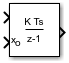### When to Use the State Port

Use the state port instead of the output port:

• When the output of the block is fed back into the block through the reset port or the initial condition port, causing an algebraic loop. For an example, see the `sldemo_bounce_two_integrators` model.

• When you want to pass the state from one conditionally executed subsystem to another, which can cause timing problems. For an example, see Building a Clutch Lock-Up Model.

You can work around these problems by passing the state through the state port rather than the output port. Simulink generates the state at a slightly different time from the output, which protects your model from these problems. To output the block state, select the Show state port check box. The state port appears on the top of the block.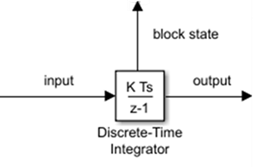### Limit the Integral

To keep the output within certain levels, select the Limit output check box and enter the limits in the corresponding text box. Doing so causes the block to function as a limited integrator. When the output reaches the limits, the integral action turns off to prevent integral windup. During a simulation, you can change the limits but you cannot change whether the output is limited. The table shows how the block determines output.

IntegralOutput
Less than or equal to the Lower saturation limit and the input is negative Held at the Lower saturation limit
Between the Lower saturation limit and the Upper saturation limit The integral
Greater than or equal to the Upper saturation limit and the input is positive Held at the Upper saturation limit

To generate a signal that indicates when the state is being limited, select the Show saturation port check box. A new saturation port appears below the block output port.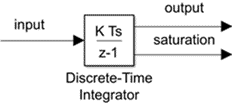The saturation signal has one of three values:

• 1 indicates that the upper limit is being applied.

• 0 indicates that the integral is not limited.

• -1 indicates that the lower limit is being applied.

### Reset the State

The block resets its state to the specified initial condition, based on an external signal. To cause the block to reset its state, select one of the External reset parameter options. A reset port appears that indicates the reset trigger type.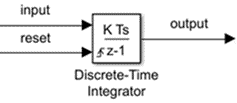The reset port has direct feedthrough. If the block output feeds back into this port, either directly or through a series of blocks with direct feedthrough, an algebraic loop results. To resolve this loop, feed the output of the block state port into the reset port instead. To access the block state, select the Show state port check box.

### Reset Trigger Types

The External reset parameter lets you determine the attribute of the reset signal that triggers the reset. The trigger options include:

• `rising` – Resets the state when the reset signal has a rising edge. For example, this figure shows the effect that a rising reset trigger has on backward Euler integration.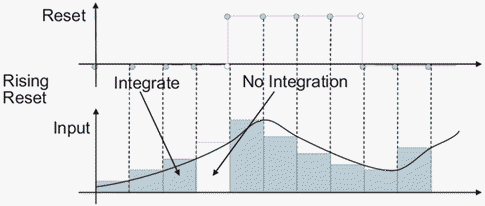• `falling` — Resets the state when the reset signal has a falling edge. For example, this figure shows the effect that a falling reset trigger has on backward Euler integration.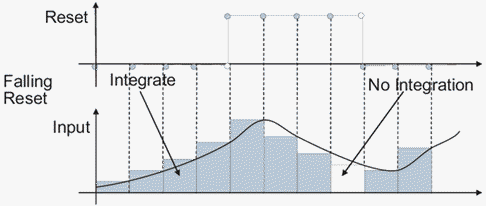• `either` — Resets the state when the reset signal rises or falls. For example, the following figure shows the effect that an either reset trigger has on backward Euler integration.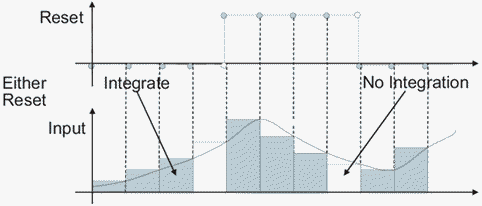• `level` — Resets and holds the output to the initial condition while the reset signal is nonzero. For example, this figure shows the effect that a level reset trigger has on backward Euler integration.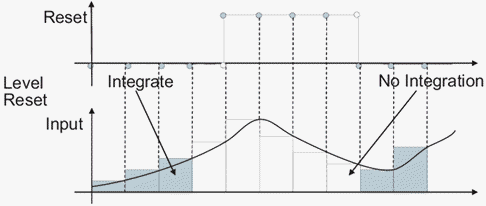• `sampled level` — Resets the output to the initial condition when the reset signal is nonzero. For example, this figure shows the effect that a sampled level reset trigger has on backward Euler integration.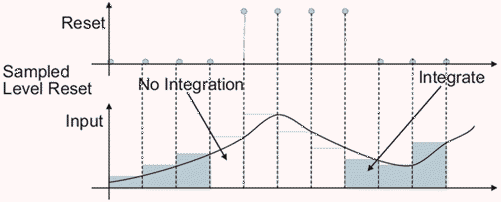The `sampled level` reset option requires fewer computations, making it more efficient than the `level` reset option.

Note

For the Discrete-Time Integrator block, all trigger detections are based on signals with positive values. For example, a signal changing from -1 to 0 is not considered a rising edge, but a signal changing from 0 to 1 is.

### Behavior in Simplified Initialization Mode

Simplified initialization mode is enabled when you set Underspecified initialization detection to `Simplified` in the Configuration Parameters dialog box. If you use simplified initialization mode, the behavior of the Discrete-Time Integrator block differs from classic initialization mode. The new initialization behavior is more robust and provides more consistent behavior in these cases:

• In algebraic loops

• On enable and disable

• When comparing results using triggered sample time against explicit sample time, where the block is triggered at the same rate as the explicit sample time

Simplified initialization mode enables easier conversion from Continuous-Time Integrator blocks to Discrete-Time Integrator blocks, because the initial conditions have the same meaning for both blocks.

For more information on classic and simplified initialization modes, see Underspecified initialization detection.

Enable and Disable Behavior with Initial Condition Setting set to Output

When you use simplified initialization mode with Initial condition setting set to `Output` for triggered and function-call subsystems, the enable and disable behavior of the block is simplified as follows.

At disable time `td`:

``` y(td) = y(td-1) ```

At enable time `te`:

• If the parent subsystem control port has States when enabling set to `reset`:

`y(te) = IC.`
• If the parent subsystem control port has States when enabling set to `held`:

`y(te) = y(td).`

The following figure shows this condition.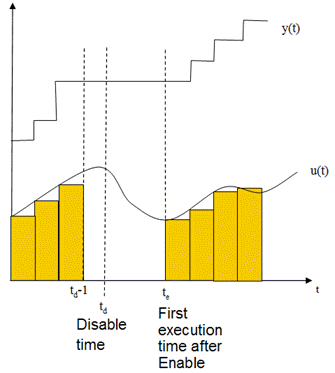Iterator Subsystems

When using simplified initialization mode, you cannot place the Discrete-Time Integrator block in an iterator subsystem block.

In simplified initialization mode, Iterator subsystems do not maintain elapsed time. Thus, if a Discrete-Time Integrator block, which needs elapsed time, is placed inside an iterator subsystem block, Simulink reports an error.

### Behavior in an Enabled Subsystem Inside a Function-Call Subsystem

Suppose you have a function-call subsystem that includes an enabled subsystem, which contains a Discrete-Time Integrator block. The following behavior applies.

Integrator MethodSample Time Type of Function-Call Trigger Port Value of `delta T` When Function-Call Subsystem Executes for the First Time After Enabled Reason for Behavior

Forward Euler

Triggered

`t — tstart`

When the function-call subsystem executes for the first time, the integrator algorithm uses `tstart` as the previous simulation time.

Backward Euler and Trapezoidal

Triggered

`t — tprevious`

When the function-call subsystem executes for the first time, the integrator algorithm uses `tprevious` as the previous simulation time.

Forward Euler, Backward Euler, and Trapezoidal

Periodic

Sample time of the function-call generator

In periodic mode, the Discrete-Time Integrator block uses sample time of the function-call generator for ```delta T```.

## Ports

### Input

expand all

Input signal, specified as a scalar, vector, or matrix

Data Types: `single` | `double` | `int8` | `int16` | `int32` | `int64` | `uint8` | `uint16` | `uint32` | `uint64` | `fixed point`

Initial conditions of the states, specified as a finite scalar, vector, or matrix.

#### Dependencies

To enable this port, set Initial condition source to `external`.

Data Types: `single` | `double` | `int8` | `int16` | `int32` | `int64` | `uint8` | `uint16` | `uint32` | `uint64` | `fixed point`

### Output

expand all

Discrete-time integration or accumulation of the input signal, specified as a scalar, vector, or matrix.

Data Types: `single` | `double` | `int8` | `int16` | `int32` | `int64` | `uint8` | `uint16` | `uint32` | `uint64` | `fixed point`

Signal indicating when the state is being limited, specified as a scalar, vector, or matrix. The signal has one of three values:

• `1` indicates that the upper limit is being applied.

• `0` indicates that the integral is not limited.

• `-1` indicates that the lower limit is being applied.

#### Dependencies

To enable this port, select the Show saturation port check box.

Data Types: `single` | `double` | `int8`

Block states, output as a scalar, vector, or matrix. By default, the block adds this port to the top of the block icon. Use the state port when:

• The output of the block is fed back into the block through the reset port or the initial condition port, causing an algebraic loop. For an example, see the `sldemo_bounce_two_integrators` model.

• You want to pass the state from one conditionally executed subsystem to another, which can cause timing problems. For an example, see the `sldemo_clutch` model.

For more information, see When to Use the State Port.

#### Dependencies

To enable this port, select the Show state port check box.

Data Types: `single` | `double` | `int8` | `int16` | `int32` | `int64` | `uint8` | `uint16` | `uint32` | `uint64` | `fixed point`

## Parameters

expand all

### Main

Specify the integration or accumulation method. See Output Equations and Integration and Accumulation Methods for more information.

#### Programmatic Use

 Block Parameter: `IntegratorMethod` Type: character vector Values: `'Integration: Forward Euler'` | `'Integration: Backward Euler'` | `'Integration: Trapezoidal'` | `'Accumulation: Forward Euler'` | `'Accumulation: Backward Euler'` | `'Accumulation: Trapezoidal'` Default: `'Integration: Forward Euler'`

Specify a scalar, vector, or matrix by which to multiply the integrator input. Each element of the gain must be a positive real number.

• Specifying a value other than 1.0 (the default) is semantically equivalent to connecting a Gain block to the input of the integrator.

• Valid entries include:

• `double(1.0)`

• `single(1.0)`

• `[1.1 2.2 3.3 4.4]`

• `[1.1 2.2; 3.3 4.4]`

Tip

Using this parameter to specify the input gain eliminates a multiplication operation in the generated code. However, this parameter must be nontunable to realize this benefit. If you want to tune the input gain, set this parameter to 1.0 and use an external Gain block to specify the input gain.

#### Programmatic Use

 Block Parameter: `gainval` Type: character vector Values: scalar | vector Default: `'1.0'`

Select the type of trigger event that resets the states to their initial conditions:

• `none` — Do not reset the state to initial conditions.

• `rising` — Reset the state when the reset signal has a rising edge.

• `falling` — Reset the state when the reset signal has a falling edge.

• `either` — Reset the state when the reset signal rises or falls.

• `level` — Reset and hold the output to the initial condition while the reset signal is nonzero.

• `sampled level` — Reset the output to the initial condition when the reset signal is nonzero.

For more information, see Reset the State and Reset Trigger Types.

#### Programmatic Use

 Block Parameter: `ExternalReset` Type: character vector Values: `'none'` | `'rising'` | `'falling'` | `'either'` | `'level'` | ```'sampled level'``` Default: `'none'`

Select source of initial condition:

• `internal` — Get the initial conditions of the states from the Initial condition block parameter.

• `external` — Get the initial conditions of the states from an external block, via the IC input port.

#### Dependencies

Selecting `internal` enables the Initial condition parameter.

Selecting `external` disables the Initial condition parameter and enables the IC input port.

#### Programmatic Use

 Block Parameter: `InitialConditionSource` Type: character vector, string Values: `'internal'` | `'external'` Default: `'internal'`

Specify initial condition of the block states. The minimum and maximum values are bound by the Output minimum and Output maximum block parameters.

Tip

Simulink software does not allow the initial condition of this block to be `inf` or `NaN`.

#### Dependencies

To enable this parameter, set the Initial condition source to `internal`.

#### Programmatic Use

 Block Parameter: `InitialCondition` Type: character vector, string Values: scalar | vector | matrix Default: `'0'`

Select whether to apply the value of the Initial condition parameter to the block state or block output. The initial condition is also the reset value.

• `Auto` — Block chooses where to apply the Initial condition parameter.

• If the block is in a non-triggered subsystem and Integrator method is set to an integration method, set initial conditions:

`x(0) = IC`

At reset:

`x(n) = IC`

• If the block is in a triggered or function-call subsystem and Integrator method is set to an integration method, set initial conditions as if `output` was selected.

• `Output` — Use this option when the block is in a triggered or a function-call subsystem and Integrator method is set to an integration method.

Set initial conditions:

`y(0) = IC`

At reset:

`y(n) = IC`

• `Compatibility` — This option is present to provide backward compatibility. You cannot select this option for Discrete-Time Integrator blocks in Simulink models but you can select it for Discrete-Time Integrator blocks in a library. Use this option to maintain compatibility with Simulink models created before R2014a.

Prior to R2014a, the option `Auto` was known as ```State only (most efficient)```. The option `Output` was known as `State and output`. The behavior of the block with the option `Compatibility` is as follows.

• If Underspecified initialization detection is set to `Classic`, the Initial condition setting parameter behaves as `Auto`.

• If Underspecified initialization detection is set to `Simplified`, the Initial condition setting parameter behaves as `Output`.

Note

This parameter was named Use initial condition as initial and reset value for in Simulink before R2014a.

#### Programmatic Use

 Block Parameter: `InitialConditionSetting` Type: character vector Value: `'Auto'` | `'Output'` | `'Compatibility'` Default: `'Auto'`

Enter the discrete time interval between steps.

By default, the block uses a discrete sample time of `1`. To set a different sample time, enter another discrete value, such as 0.1.

See Specify Sample Time for more information.

#### Tips

• Do not specify a sample time of 0. This value specifies a continuous sample time, which the Discrete-Time Integrator block does not support.

• Do not specify a sample time of `inf` or `NaN` because these values are not discrete.

• If you specify -1 to inherit the sample time from an upstream block, verify that the upstream block uses a discrete sample time. For example, the Discrete-Time Integrator block cannot inherit a sample time of 0.

#### Programmatic Use

 Block Parameter: `SampleTime` Type: character vector Values: scalar | vector Default: `'-1'`

Limit the block's output to a value between the Lower saturation limit and Upper saturation limit parameters.

• Selecting this check box limits the block's output to a value between the Lower saturation limit and Upper saturation limit parameters.

• Clearing this check box does not limit the block's output values.

#### Dependencies

Selecting this parameter enables the Lower saturation limit and Upper saturation limit parameters.

#### Programmatic Use

 Block Parameter: `LimitOutput` Type: character vector Values: `'off'` | `'on'` Default: `'off'`

Specify the upper limit for the integral as a scalar, vector, or matrix. You must specify a value between the Output minimum and Output maximum parameter values.

#### Dependencies

To enable this parameter, select the Limit output check box.

#### Programmatic Use

 Block Parameter: `UpperSaturationLimit` Type: character vector, string Values: scalar | vector | matrix Default: `'inf'`

Specify the lower limit for the integral as a scalar, vector, or matrix. You must specify a value between the Output minimum and Output maximum parameter values.

#### Dependencies

To enable this parameter, select the Limit output check box.

#### Programmatic Use

 Block Parameter: `LowerSaturationLimit` Type: character vector , string Values: scalar | vector | matrix Default: `'-inf'`

Select this check box to add a saturation output port to the block. When you clear this check box, the block does not have a saturation output port.

#### Dependencies

Selecting this parameter enables a saturation output port.

#### Programmatic Use

 Block Parameter: `ShowSaturationPort` Type: character vector , string Values: `'off'` | `'on'` Default: `'off'`

Select this check box to add a state output port to the block. When you clear this check box, the block does not have a state output port.

#### Dependencies

Selecting this parameter enables a state output port.

#### Programmatic Use

 Block Parameter: `ShowStatePort` Type: character vector , string Values: `'off'` | `'on'` Default: `'off'`

Select this check box to have Simulink linearization commands treat this block as not resettable and as having no limits on its output, regardless of the settings of the block reset and output limitation options.

Tip

Ignoring the limit and resetting allows you to linearize a model around an operating point. This point may cause the integrator to reset or saturate.

#### Programmatic Use

 Block Parameter: `IgnoreLimit` Type: character vector Values: `'off'` | `'on'` Default: `'off'`

### Signal Attributes

Lower value of the output range that Simulink checks.

Simulink uses the minimum to perform:

Note

Output minimum does not saturate or clip the actual output signal. Use the Saturation block instead.

#### Programmatic Use

 Block Parameter: `OutMin` Type: character vector Values: `'[ ]'`| scalar Default: `'[ ]'`

Upper value of the output range that Simulink checks.

Simulink uses the maximum value to perform:

Note

Output maximum does not saturate or clip the actual output signal. Use the Saturation block instead.

#### Programmatic Use

 Block Parameter: `OutMax` Type: character vector Values: `'[ ]'`| scalar Default: `'[ ]'`

Choose the data type for the output. The type can be inherited, specified directly, or expressed as a data type object such as `Simulink.NumericType`. For more information, see Control Data Types of Signals.

When you select an inherited option, the block behaves as follows:

• `Inherit: Inherit via internal rule` — Simulink chooses a data type to balance numerical accuracy, performance, and generated code size, while taking into account the properties of the embedded target hardware. If you change the embedded target settings, the data type selected by the internal rule might change. For example, if the block multiplies an input of type `int8` by a gain of `int16` and `ASIC/FPGA` is specified as the targeted hardware type, the output data type is `sfix24`. If `Unspecified (assume 32-bit Generic)`, i.e., a generic 32-bit microprocessor, is specified as the target hardware, the output data type is `int32`. If none of the word lengths provided by the target microprocessor can accommodate the output range, Simulink software displays an error in the Diagnostic Viewer.

It is not always possible for the software to optimize code efficiency and numerical accuracy at the same time. If the internal rule doesn’t meet your specific needs for numerical accuracy or performance, use one of the following options:

• Specify the output data type explicitly.

• Use the simple choice of `Inherit: Same as input`.

• Explicitly specify a default data type such as `fixdt(1,32,16)` and then use the Fixed-Point Tool to propose data types for your model. For more information, see `fxptdlg` (Fixed-Point Designer).

• To specify your own inheritance rule, use `Inherit: Inherit via back propagation` and then use a Data Type Propagation block. Examples of how to use this block are available in the Signal Attributes library Data Type Propagation Examples block.

• `Inherit: Inherit via back propagation` — Use data type of the driving block.

#### Programmatic Use

 Block Parameter: `OutDataTypeStr` Type: character vector Values: ```'Inherit: Inherit via internal rule``` | `'Inherit: Inherit via back propagation'` | `'double'` | `'single'` | `'int8'` | `'uint8'` | `'int16'` | `'uint16'` | `'int32'` | `'uint32'` | `'int64'` | `'uint64'` | `'fixdt(1,16)'` | `'fixdt(1,16,0)'` | `'fixdt(1,16,2^0,0)'` | ```''``` Default: `'Inherit: Inherit via internal rule'`

Select this parameter to prevent the fixed-point tools from overriding the Output data type you specify on the block. For more information, see Use Lock Output Data Type Setting (Fixed-Point Designer).

#### Programmatic Use

 Block Parameter: `LockScale` Type: character vector Values: `'off' | 'on'` Default: `'off'`

Choose one of these rounding modes.

`Ceiling`

Rounds both positive and negative numbers toward positive infinity. Equivalent to the MATLAB® `ceil` function.

`Convergent`

Rounds number to the nearest representable value. If a tie occurs, rounds to the nearest even integer. Equivalent to the Fixed-Point Designer™ `convergent` function.

`Floor`

Rounds both positive and negative numbers toward negative infinity. Equivalent to the MATLAB `floor` function.

`Nearest`

Rounds number to the nearest representable value. If a tie occurs, rounds toward positive infinity. Equivalent to the Fixed-Point Designer `nearest` function.

`Round`

Rounds number to the nearest representable value. If a tie occurs, rounds positive numbers toward positive infinity and rounds negative numbers toward negative infinity. Equivalent to the Fixed-Point Designer `round` function.

`Simplest`

Automatically chooses between round toward floor and round toward zero to generate rounding code that is as efficient as possible.

`Zero`

Rounds number toward zero. Equivalent to the MATLAB `fix` function.

#### Programmatic Use

 Block Parameter: `RndMeth` Type: character vector Values: `'Ceiling'` | `'Convergent'` | `'Floor'` | `'Nearest'` | `'Round'` | `'Simplest'` | `'Zero'` Default: `'Floor'`

For more information, see Rounding (Fixed-Point Designer).

Specify whether overflows saturate or wrap.

• `off` — Overflows wrap to the appropriate value that the data type can represent.

For example, the number 130 does not fit in a signed 8-bit integer and wraps to -126.

• `on` — Overflows saturate to either the minimum or maximum value that the data type can represent.

For example, an overflow associated with a signed 8-bit integer can saturate to -128 or 127.

Tip

• Consider selecting this check box when your model has a possible overflow and you want explicit saturation protection in the generated code.

• Consider clearing this check box when you want to optimize efficiency of your generated code.

Clearing this check box also helps you to avoid overspecifying how a block handles out-of-range signals. For more information, see Troubleshoot Signal Range Errors.

• When you select this check box, saturation applies to every internal operation on the block, not just the output or result.

• In general, the code generation process can detect when overflow is not possible. In this case, the code generator does not produce saturation code.

#### Programmatic Use

 Block Parameter: `SaturateOnIntegerOverflow` Type: character vector Values: `'off' | 'on'` Default: `'off'`

### State Attributes

Use this parameter to assign a unique name to the block state. The default is `' '`. When this field is blank, no name is assigned. When using this parameter, remember these considerations:

• A valid identifier starts with an alphabetic or underscore character, followed by alphanumeric or underscore characters.

• The state name applies only to the selected block.

For more information, see C Code Generation Configuration for Model Interface Elements (Simulink Coder).

#### Dependencies

When you specify a value for State name and click , you enable the State name must resolve to Simulink signal object parameter.

#### Programmatic Use

 Parameter: `StateName` Type: character vector Values: unique name Default: `''`

Specify whether state names are required to resolve to signal objects. If selected, the software generates an error at run time if you specify a state name that does not match the name of a signal object.

Selecting this parameter disables the Code generation storage class parameter.

#### Dependencies

Enabled when you specify a value for the State name parameter and set the Signal resolution model configuration parameter to a value other than `None`.

#### Programmatic Use

 Block Parameter: `StateMustResolveToSignalObject` Type: character vector Values: `'off'` | `'on'` Default: `'off'`

## Block Characteristics

 Data Types `double` | `fixed point` | `integer` | `single` Direct Feedthrough `yes` Multidimensional Signals `no` Variable-Size Signals `no` Zero-Crossing Detection `no`

## Version History

Introduced before R2006a